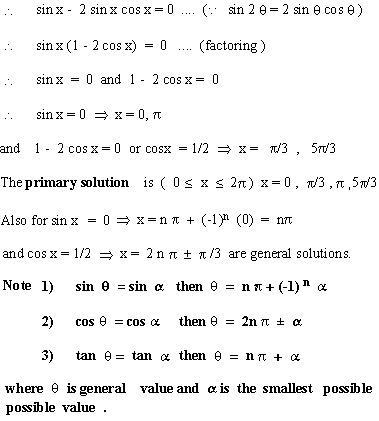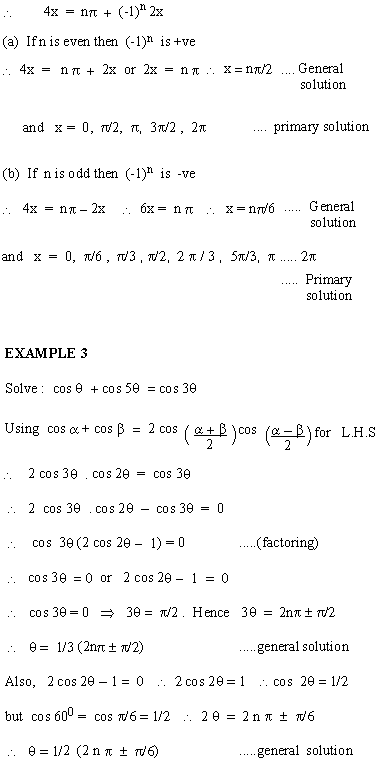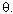Support the Monkey! Tell All your Friends and TeachersHome MonkeyNotes Printable Notes Digital Library Study Guides Study Smart Parents Tips College Planning Test Prep Fun Zone Help / FAQ How to Cite New Title Request

 6.2 TRIGONOMETRIC EQUATIONS Equations involving trigonometric functions of unknown angles are called 1) identities, if they are satisfied by all values of the unknown angles for which the trigonometric functions are defined. 2) Conditional equations which are satisfied by particular values of the unknown angles. A Solution is a value of the angle which satisfies a given trigonometric equation. Solution in a particular interval, such as 0 £ x £ 2p are usually known as "primary solution". A general solution is that formula which lists all possible solutions. No clear - cut procedure of solving general trigonometric equation exist. It involves the use of identities, algebraic manipulation, trial and error etc. Some standard procedures are given below. 1) Factoring (2) Squaring both sides (3) Expressing various functions in terms of single function . Example 1 Solve sin x - sin 2x = 0 Solution : sin x - sin 2x = 0Example 2 Solve : sin 4x - sin 2x = 0 Solution : sin 4x - sin 2x = 0 sin 4x = sin 2xNow putting n = 0, 1, 2, ... the values ofin the range 0 ££ 2 p gives the primary solution. Index 6.1 Inverse Functions 6.2 Trignometric Equations Supplementary Problems Chapter 7
 Search: All Products Books Popular Music Classical Music Video DVD Toys & Games Electronics Software Tools & Hardware Outdoor Living Kitchen & Housewares Camera & Photo Cell Phones Keywords: